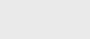2年前 (2021-10-23) |   抢沙发  2760

[收起] 文章目录

## ==  还有！= 运算符

### 原语

``assertThat(1 == 1).isTrue();``

``````Integer a = new Integer(1);
assertThat(1 == a).isTrue();``````

### Objects

``````Integer a = new Integer(1);
Integer b = new Integer(1);

assertThat(a == b).isFalse();``````

``````Integer a = new Integer(1);
Integer b = a;

assertThat(a == b).isTrue();``````

``````Integer a = Integer.valueOf(1);
Integer b = Integer.valueOf(1);

assertThat(a == b).isTrue();``````

Java也对字符串执行此操作：

``````assertThat("Hello!" == "Hello!").isTrue();
``````

``````assertThat(null == null).isTrue();

assertThat("Hello!" == null).isFalse();``````

### Object#equals Method

``````Integer a = new Integer(1);
Integer b = new Integer(1);

assertThat(a.equals(b)).isTrue();
``````

``````public class Person {
private String firstName;
private String lastName;

public Person(String firstName, String lastName) {
this.firstName = firstName;
this.lastName = lastName;
}
}``````

``````@Override
public boolean equals(Object o) {
if (this == o) return true;
if (o == null || getClass() != o.getClass()) return false;
Person that = (Person) o;
return firstName.equals(that.firstName) &&
lastName.equals(that.lastName);
}``````

### Objects#equals静态方法

`Objects`助手类的`equals（）`方法解决了这个问题。它接受两个参数并对它们进行比较，还处理空值。

``````Person joe = new Person("Joe", "Portman");
Person joeAgain = new Person("Joe", "Portman");
Person natalie = new Person("Natalie", "Portman");

assertThat(Objects.equals(joe, joeAgain)).isTrue();
assertThat(Objects.equals(joe, natalie)).isFalse();``````

``````public Person(String firstName, String lastName, LocalDate birthDate) {
this(firstName, lastName);
this.birthDate = birthDate;
}``````

``birthDate == null ? that.birthDate == null : birthDate.equals(that.birthDate);``

``Objects.equals(birthDate, that.birthDate);``

### Comparable接口

`Compariable`接口是泛型接口，只有一个方法`compareTo（）`，该方法接受泛型类型的参数并返回`int`。如果该参数小于该参数，则返回负值；如果它们相等，则返回`0`；否则返回正值。

``````public class Person implements Comparable<Person> {
//...

@Override
public int compareTo(Person o) {
return this.lastName.compareTo(o.lastName);
}
}``````

### Comparator接口

`Comparator`接口是泛型的，并且有一个`compare`方法，该方法接受该泛型类型的两个参数并返回一个整数。我们在前面已经看到了类似接口的模式。

Comparator相似；但是，它与类的定义是分开的。因此，我们可以为一个类定义任意多个`Comparator`，其中我们只能提供一个可比较的实现。

``````Comparator<Person> compareByFirstNames = Comparator.comparing(Person::getFirstName);
``````

``````Person joe = new Person("Joe", "Portman");
Person allan = new Person("Allan", "Dale");

List<Person> people = new ArrayList<>();

people.sort(compareByFirstNames);

assertThat(people).containsExactly(allan, joe);``````

`Comparator`接口上还有其他方法可用于`compareTo（）`实现：

``````@Override
public int compareTo(Person o) {
return Comparator.comparing(Person::getLastName)
.thenComparing(Person::getFirstName)
.thenComparing(Person::getBirthDate, Comparator.nullsLast(Comparator.naturalOrder()))
.compare(this, o);
}``````

### Apache Commons

``````<dependency>
<groupId>org.apache.commons</groupId>
<artifactId>commons-lang3</artifactId>
<version>3.12.0</version>
</dependency>``````

### ObjectUtils#notEqual方法

``````String a = new String("Hello!");
String b = new String("Hello World!");

assertThat(ObjectUtils.notEqual(a, b)).isTrue();``````

### ObjectUtils#compare方法

``````String first = new String("Hello!");
String second = new String("How are you?");

assertThat(ObjectUtils.compare(first, second)).isNegative();
``````

### Guava

``````<dependency>
<artifactId>guava</artifactId>
<version>29.0-jre</version>
</dependency>``````

#### Objects#equal方法

``````String a = new String("Hello!");
String b = new String("Hello!");

assertThat(Objects.equal(a, b)).isTrue();``````

#### Comparison方法

``assertThat(Ints.compare(1, 2)).isNegative();``

#### ComparisonChain类

``````Person natalie = new Person("Natalie", "Portman");
Person joe = new Person("Joe", "Portman");

int comparisonResult = ComparisonChain.start()
.compare(natalie.getLastName(), joe.getLastName())
.compare(natalie.getFirstName(), joe.getFirstName())
.result();

assertThat(comparisonResult).isPositive();
``````

## 结论### 注册